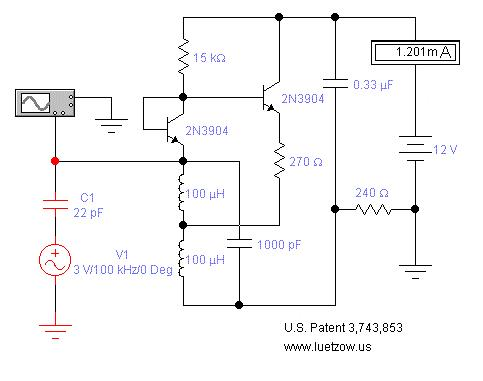Making a SPICE simulated electronic oscillator circuit oscillate on the first try.
The oscillator circuit shown in Figure One uses an AC Voltage Source V1 and Capacitor C1 to inject a
small ac signal to kick start the circuit's oscillation function into action.  I have observe that, when both
Electronic WorkBench 5.12 and Multisim 7 are used to simulate an electronic oscillator circuit, the
circuit will sometime refuse to show any sign of an oscillation function.  But, when a circuit is kick
started into oscillation the first time using V1 and C1, it will continue to oscillate when V1 and C1 are
removed from the circuit as long as the spice circuit file is not deleted from the computer.

It is very easy to design an oscillator circuit and make it oscillate using V1 and C1.  First, find the
oscillator circuit you want to simulate and load the schematic into the spice simulator.  If you have
copied the circuit from a U. S. Patent and there are no part values, select the oscillating frequency and
pick the inductance and capacitance values needed to resonate the tank circuit at that frequency. Next,
select the transistor required for the circuit.  A 2N3904 is a good starting point for a NPN transistor
and a 2N3906 is ok for a PNP transistor. In Figure One, the emitter resistor will be a low resistance
value and a value in the area of 270 Ohms is ok.  A 15K resistor is a nice selection for the base bias
resistor.  C1 can be any value form 10 pF to 50 pF. The frequency of V1 should be some where at or
below the resonant frequency of the oscillator with a voltage level of 3 to 5 Volts.

Once you have the circuit oscillating, lower the value of C1 and readjust the oscillator circuit part
values, if needed,  to keep it oscillating.  When you have the value of C1 down to 1 pF, you can delete
V1 and C1 form the circuit.  Next you should adjust the circuit components for proper circuit functions
such DC current draw, transistor dissipation, and oscillator output.
Figure One: A simple oscillator circuit that uses V1 and C1 to assist in
starting the initial electronic oscillation function of the circuit.